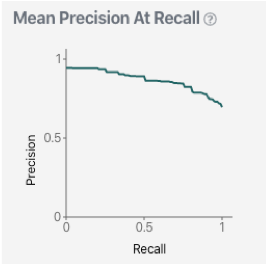# Precision

Precision measures the proportion of the predictions made by the model that were actually correct. That is, of all the positive predictions that the model made, what proportion were true positives.

Precision =                         true positives

true positives + false positives

For example, for every 100 verbatims in a dataset predicted as having the ‘Request for information’ label, the precision is the percentage of times that the ‘Request for information’ was correctly predicted out of the total times it was predicted.

A 95% precision would mean that for every 100 verbatims predicted as having a specific label, 95 would be correctly labelled, and 5 would be wrongly labelled (i.e. they should not have been labelled with that label).

For a more detailed explanation on how precision works, please see here.

Average Precision (AP)

The average precision (AP) score for an individual label is calculated as the average of all the precision scores at each recall value (between 0 and 100%) for that label.

Essentially, the average precision measures how well the model performs across all confidence thresholds for that label.

Mean average precision (MAP)

Mean average precision (MAP) is one of the most useful measures of overall model performance and is an easy way to compare different model versions against one another.

The MAP score takes the mean of the average precision score for every label in your taxonomy that has at least 20 examples in the training set used in Validation.

Typically, the higher the MAP score, the better the model is performing overall, though this is not the only factor that should be considered when understanding how healthy a model is. It is also important to know that your model is unbiased and has high coverage.

Mean precision at recall

The mean precision at recall is another metric that shows the overall model performance. It is presented graphically as a precision at recall curve averaged over all of the labels in your taxonomy.Example mean precision at recall curve

Previous: Organisations     |     Next: Recall

Did you find it helpful? Yes No

Send feedback